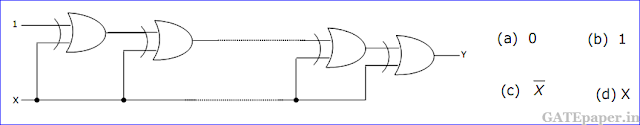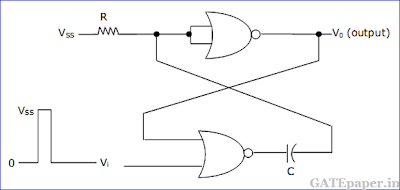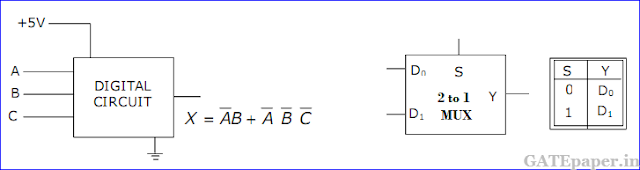### GATE 2002 ECE Video Solution on Digital Circuits (Digital Electronics)

1. 4 – bit 2’s complement representation of a decimal number is 1000. The number is
a. +8
b. 0
c. -7
d. -8

2. If the input to the digital circuit of the figure, consisting of a cascade of 20 XOR gates is X, then the output Y is equal to3. The number of comparators required in a 3 bit comparator type ADC is
a. 2
b. 3
c. 7
d. 8

4. The gates G1 and G2 in the figure is have propagation delays of 10 ns and 20 ns respectively. If the input Vi makes an abrupt change from logic 0 to 1 at time t = to, then the output waveform Vo is5. The circuit in the figure is ahs two CMOS NOR gates. This circuit functions as aa. Flip-flop
b. Schmitt trigger
c. Monostable multivibrator
d. Astable multivibrator

6. If the inputs X3, X2, X1, X0 to the ROM in the figure are 8-4-2-1 BCD numbers, then the outputs Y3Y2Y1Y0 area. Gary code numbers
b. 2-4-2-1 BCD numbers
c. Excess-3 code numbers
d. None of the above

7. Consider the following assembly language program.
MVI B, 87H
MOV A, B
START: JMP NEXT
MVI B, 00H
XRA B
OUT PORT1
HLT
NEXT: XRA B
JP START
OUT PORT2
HLT
The execution of the above program in an 8085 microprocessor will result in
a. An output of 87H at PORT1
b. An output of 87H at PORT2
c. Infinite looping of the program execution with accumulator data remaining at 00H
d. Infinite looping of the program execution with accumulator data alternating between 00H and 87H

8. The inputs to a digital circuit shown in figure is are the external signals A, B and C. Assume complements of A, B and C are not available. The +5 volts power supply (logic 1) and the ground (logic 0) are also available.a. Write down the output function in its canonical SOP and POS forms.
b. Implement the circuit using only 2 to 1 multiplexers shown in the figure, where S is the data select line, D0 and D1 are the input data lines and Y is the output line. The function table is also given for the multiplexer.

9. It is required to design a binary mod-5 synchronous counter using AB flip-flop, such that the output Q2Q1Q0 changes as 000 -> 001 -> 010..... and so on. The truth table for the AB flip-flop is given in figure.a. Write down the state table for the mod-5 counter
b. Obtain simplified SOP expressions for the inputs A2, B2, A1, B1, A0 and B0 in terms of Q2, Q1, Q0 and their complements.
c. Hence complete the circuit diagram for the mod-5 counter given in the figure using minimum number of 2 input NAND gate only.

10. An 8085 microprocessor operating at 5 MHz clock frequency executes the following routine.
START: MOV A, B
OUT 55H
DCR B
STA FFF8H
JNP START
a. Determine the total number of machine cycles required to execute the routine till the JMP instruction is executed for the first time.
b. Determine the time interval between two consecutive (MEMW)’ signals.
c. If the external logic controls the READSY line so that three WAIT states are introduced in the I/O WRITE machine cycle, determine the time interval between two consecutive (MEMW)’ signals.

Solution :